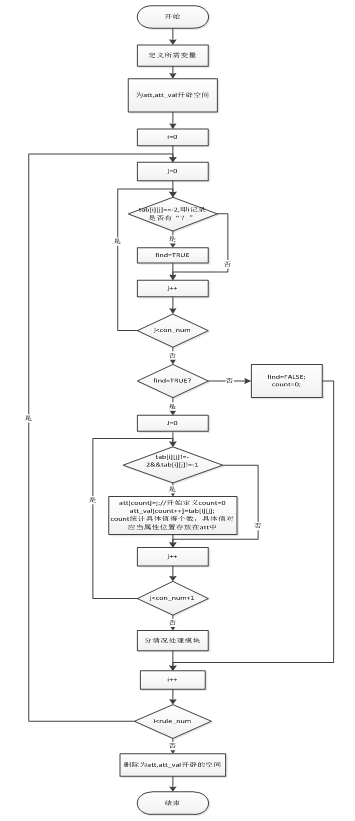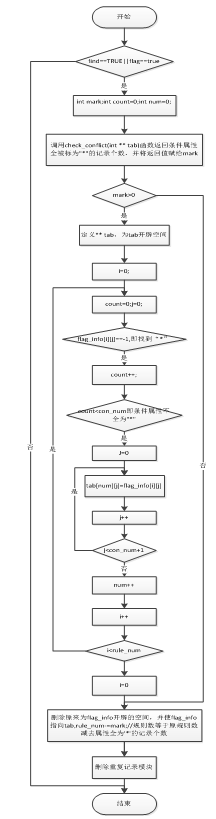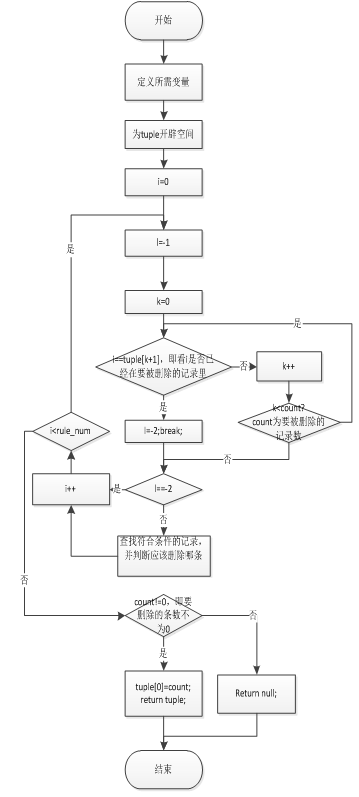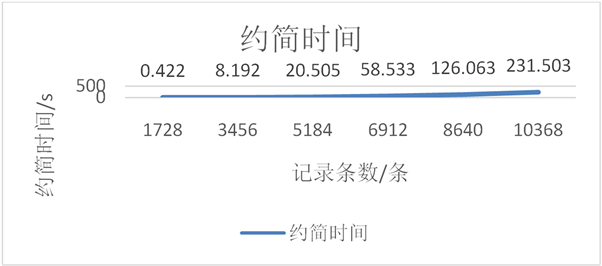﻿ 启发式值约简算法的研究与实现

# 启发式值约简算法的研究与实现The Study and Implementation of Heuristic Value Reduction

Abstract: Based on the research of rough set theory, this paper studies the process of heuristic value reduction. It usually constructs the decision table composed of the reduced attribute set and the decision attribute. Then, the heuristic information is used to perform the de-operation and delete the duplicate information. Finally, the new decision table works as the initial decision table and heuristic algorithm is used to judge whether the attribute values in the records are redundant or necessary. The redundant attribute values are deleted and the attribute values of the records are reduced to get the approximate minimum rule set. At last the test system is implemented.

1. 引言

2. 粗糙集基本概念

2.1. 信息表和决策表

$S=\left(U,V,A,f\right)$ 为一个信息表  ，其中U为论域，是一非空有限对象集，即 $U=\left\{x1,x2,\cdots ,xn\right\}$$A=\left\{a1,a2,\cdots ,an\right\}$ 是非空有限的属性集合；Va是属性a的值域，即 $V=\cup Va$$f:U×A\to V$ 成为信息函数，使得对每一 $a\in A$$x\in U$ ，有 $f\left(x,a\right)\in Va$ 。在粗糙集理论中，信息表可简化 $S=\left(U,A\right)$$S=\left(U,A,V\right)$

2.2. 知识和不可分辨关系

2.3. 约简和核

$Q\subseteq P$ ，如果Q是独立的，且 $ind\left(Q\right)=ind\left(P\right)$ ，则称Q为P的一个约简。显然，P可以由多个约简。P中所有的必要属性组成的集合称为P的核，记作core(P)。

2.4. 值约简相关概念Table 1. An instance of core attributes based decision rule

$DRC\left(y\right)=\left\{{d}_{x}:des\left({\left[x\right]}_{C}\right)⇒des\left({\left[x\right]}_{D}\right)|x\in U且{\left[x\right]}_{C}\subseteq y\right\}$$\forall y\in U/D$

$core\left(y\right)\subseteq C$$core\left({d}_{x}\right)\subseteq C$ ，且 $core\left(y\right)=\underset{{d}_{x}\in DRC\left(y\right)}{\cup }core\left({d}_{x}\right)$

3. 启发式值约简算法

3.1. 算法步骤

For(j = 1 To m-1)

For(i = 1 To n) {

If ${\exists }_{k}\left(k\ne i\wedge {\forall }_{l}\left(\left(l\ne j\wedge l\ne m\wedge {{T}^{\prime }}_{il}\ne \ast \wedge {{T}^{\prime }}_{il}\ne ?\right)\to {T}_{il}=={T}_{kl}\right)\wedge {T}_{im}\ne {T}_{km}\right)$

${{T}^{\prime }}_{ij}={T}_{ij}$ ;

Else if ${\exists }_{k}\left(k\ne i\wedge {\forall }_{l}\left(l\ne j\wedge {{T}^{\prime }}_{il}\ne \ast \wedge {{T}^{\prime }}_{il}\ne ?\to {T}_{il}=={T}_{kl}\right)\right)$

${{T}^{\prime }}_{ij}=\ast$ ;

Else ${{T}^{\prime }}_{ij}=?$ ;

}

For(i=1 To n) ${{T}^{\prime }}_{im}={T}_{im}$ ;

For(j=1 To m-1)

For(i=1 To n) {

If ${{T}^{\prime }}_{ij}==?$ {

If ${\forall }_{l}\left(l\ne m\to \left({{T}^{\prime }}_{il}==?\text{\hspace{0.17em}}\vee {{T}^{\prime }}_{il}==\ast \right)\right)$

${{T}^{\prime }}_{ij}={T}_{ij}$ ;

Else If ${\forall }_{k}\left({\forall }_{l}\left(l\ne m\wedge {{T}^{\prime }}_{il}\ne ?\wedge {{T}^{\prime }}_{il}\ne \ast \to {T}_{il}=={T}_{kl}\right)\to {T}_{im}=={T}_{km}\right)$

${{T}^{\prime }}_{ij}=\ast$ ;

Else ${{T}^{\prime }}_{ij}={T}_{ij}$ ;

}

}

For each tuple (i) in T'{

If ${\exists }_{k}{\exists }_{l}\left(l\ne m\wedge {{T}^{\prime }}_{il}\ne {{T}^{\prime }}_{kl}\wedge {{T}^{\prime }}_{il}==\ast \wedge {\forall }_{j}\left(j\ne l\to {{T}^{\prime }}_{ij}=={{T}^{\prime }}_{kj}\right)\right)$ {

If ${\forall }_{h}\left({\forall }_{j}\left(\left(j\ne m\wedge {{T}^{\prime }}_{ij}\ne \ast \right)\to {T}_{hj}=={{T}^{\prime }}_{ij}\right)\to {T}_{hm}=={{T}^{\prime }}_{im}\right)$

Else 删除记录i;

}

Else If ${\exists }_{k}{\exists }_{l}\left(l\ne m\wedge {{T}^{\prime }}_{il}\ne {{T}^{\prime }}_{kl}\wedge {{T}^{\prime }}_{kl}==\ast \wedge {\forall }_{j}\left(j\ne l\to {{T}^{\prime }}_{ij}=={{T}^{\prime }}_{kj}\right)\right)$ {

If ${\forall }_{h}\left({\forall }_{j}\left(\left(j\ne m\wedge {{T}^{\prime }}_{kj}\ne \ast \right)\to {T}_{hj}=={{T}^{\prime }}_{kj}\right)\to {T}_{hm}=={{T}^{\prime }}_{km}\right)$

Else 删除记录k;

}

}

3.2. 算法实现Figure 1. Diagram of deleting attributeFigure 2. Diagram of deleting record “?”Figure 3. Diagram of deleting record “*”Figure 4. The diagram of deleting record which is * and has only one different attribute

4. 结果分析

4.1. 简单例子

A: buying (购买价格): vhigh, high, med, low. (4, 3, 2, 1)

B: maint (维修价格): vhigh, high, med, low. (4, 3, 2, 1)

C: doors (车门数量): 2, 3, 4, 5more. (2, 3, 4, 5)

D: persons (车载人数): 2, 4, more. (2, 4, 5)

E: lug_boot (汽车后备箱型号): small, med, big. (1, 2, 3)

F: safety (安全性): low, med, high. (1, 2, 3)

G: class values (车辆价值): unacc, acc, good, vgood (1, 2, 3, 4）

1) 冲突记录----保存原该属性值；

2) 重复记录----将该属性值标记为“*”；

3) 其他剩余记录----将该属性值标记为“？”。

1) 所有条件属性均被标记----标记“？”改为原属性值；

2) 能只根据没有被标记的条件属性值就判断出决策属性值----标记“？”改为“*”；

3) 不能判断出决策----标记“？”改为原属性值。

1) 根据未被标记的属性值就能得到决策属性值----删除另一条记录；

2) 不能判断出决策属性值----删除本记录。Table 4. The reduction result of second and third stepTable 6. The comparison of different size data of heuristic value reductionFigure 5. The reduction time comparison of different data size

4.2. 性能分析

5. 总结与展望

 张文修, 吴伟志, 梁吉业, 李德玉.粗糙集理论与方法第一版[M]. 北京科学出版社, 2001.

 罗秋瑾, 陈世联. 基于值约简和决策树的最简规则提取算法[J]. 计算机应用, 2005, 25(8): 141-143.

 林嘉宜, 彭宏, 郑启伦. 一种新的基于粗糙集的值约简算法[J]. 计算机工程, 2003, 29(4): 71-129.

 杨振峰, 郭景峰, 常峰. 一种基于粗集的值约简方法[J]. 计算机工程, 2003, 29(9): 96-97.

 刘艳丽, 王海涌, 郑丽英. 基于粗集理论的决策规则约简算法的研究与应用[J]. 兰州交通大学学报(自然科学版), 2004, 23(6): 78-111.

 叶明凤. 基于核值的决策规则算法的研究[J]. 煤炭技术, 2014, 33(3): 257-259.

 林嘉宜, 彭宏, 郑启伦. 一种新的基于粗糙集的值约简算法[J]. 计算机工程, 2003(4):70-7l.

 王珍, 余昭平. 一种基于粗糙集的最小约简算法[J]. 微计算机信息, 2006(22): 218-220.

 王清毅, 范焱, 蔡庆生. 知识的约简研究[J]. 小型微型计算机系统, 2000, 21(6): 623-627.

 顾军华, 周艳聪, 宋洁, 晏俊秋.一种新的求解属性值约简算法[J]. 南开大学学报(自然科学版), 2003, 36(4): 38-42.

Top